﻿• 价格透明
• 信息保密
• 进度掌控
• 售后无忧# Machine Learning —— Semi-supervised Learning

## Introduction

Supervised Learning： ( x r , y ^ r ) r = 1 R (x^r,\hat{y}^r)^R_{r=1}

• training data中，每一组data都有input 和对应的output

Semi-supervised Learning： ( x r , y ^ r ) r = 1 R + ( x u ) u = R R + U {(x^r,\hat{y}^r)^R_{r=1}+({x^u})^{R+U}_{u=R}}

• training data中，部分data没有标签
• 无标签的数据量远大于有标签的数据量
• semi-supervised learning分为以下两种情况：
• Transductive Learning：unlabeled data is the testing data，把testing data当做无标签的training data使用，适用于事先已经知道testing data的情况
• Inductive Learning：unlabeled data is not the testing data，不把testing data的feature拿去给机器训练，适用于事先并不知道testing data的情况

## Why semi-supervised learning help？

The distribution of the unlabeled data tell us something.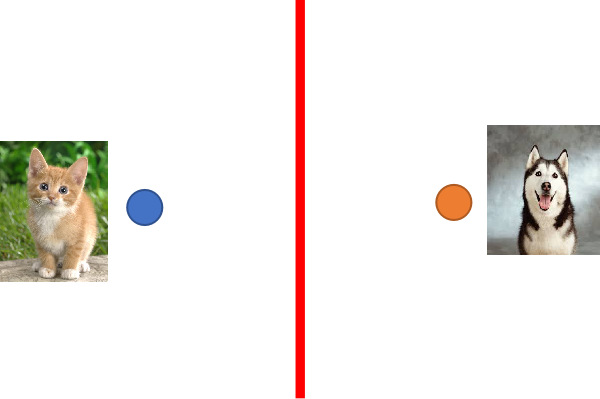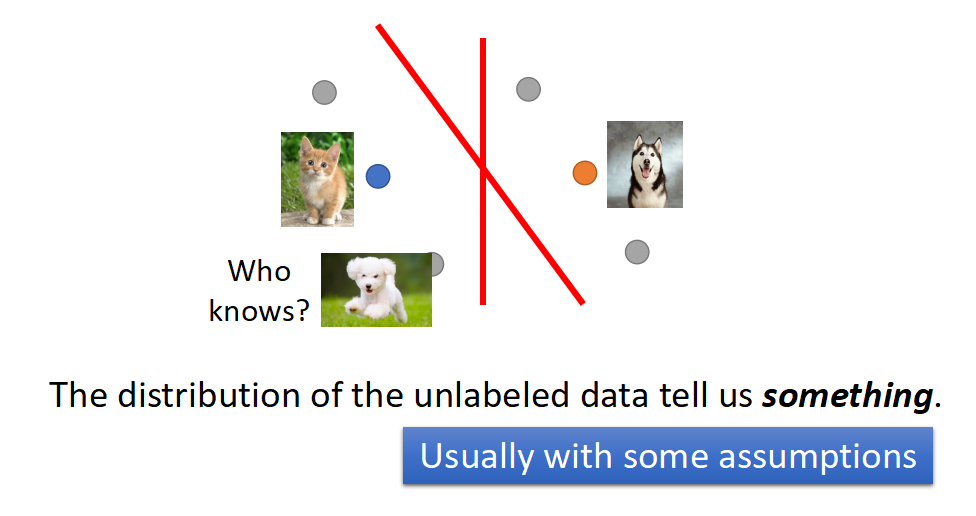semi-supervised learning的使用往往伴随着假设，而该假设的合理与否，决定了结果的好坏程度；比如上图中的unlabeled data，它显然是一只狗，而特征分布却与猫被划分在了一起，很可能是由于这两张图片的背景都是绿色导致的，因此假设是否合理显得至关重要

## Semi-supervised Learning for Generative Model

### Supervised Generative Model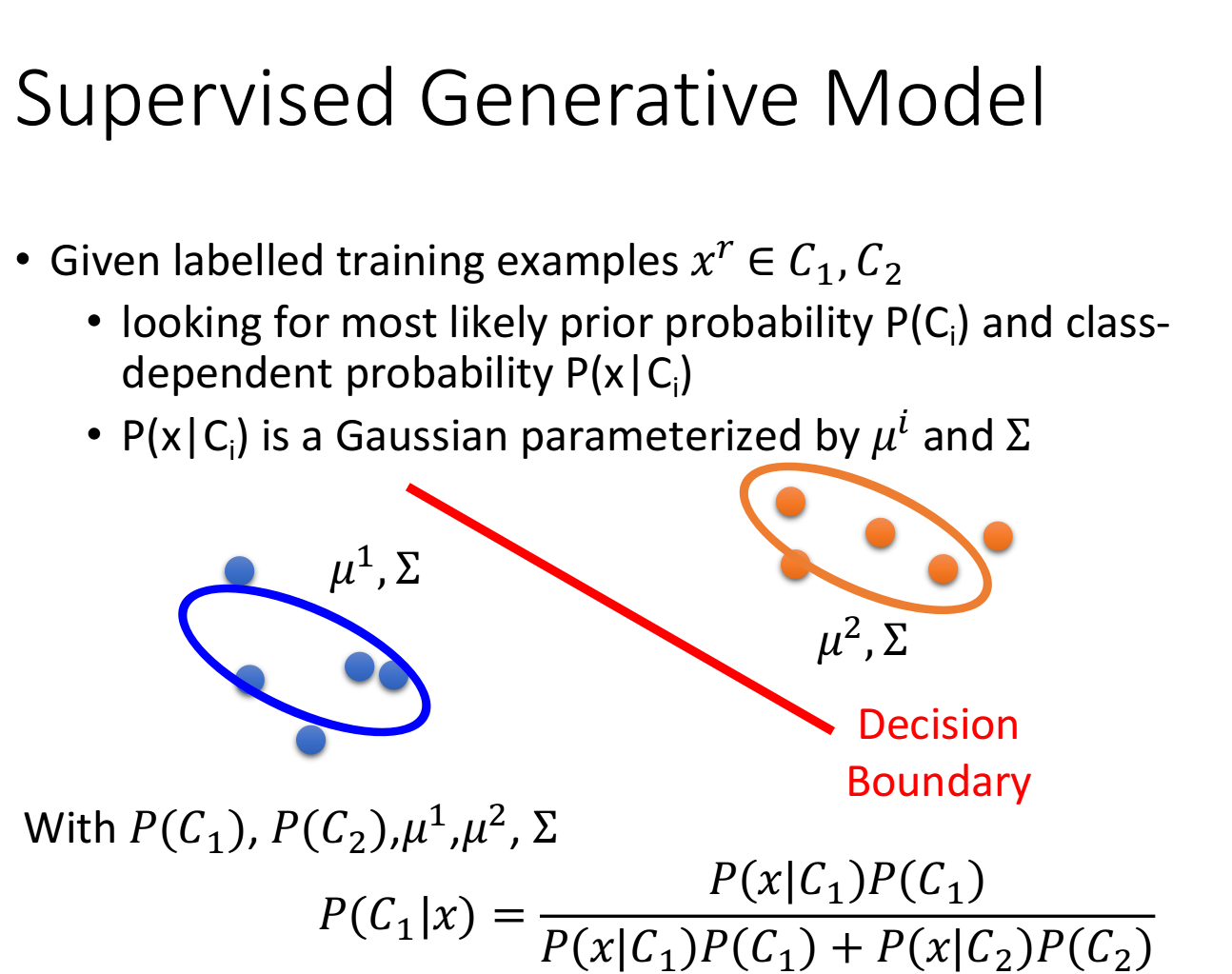### Semi-supervised Generative Model

unlabeled data对 u 1 u^1 u 2 u^2 ∑ \sum 都有一定的影响，因此划分两个class的decision boundary也会随之改变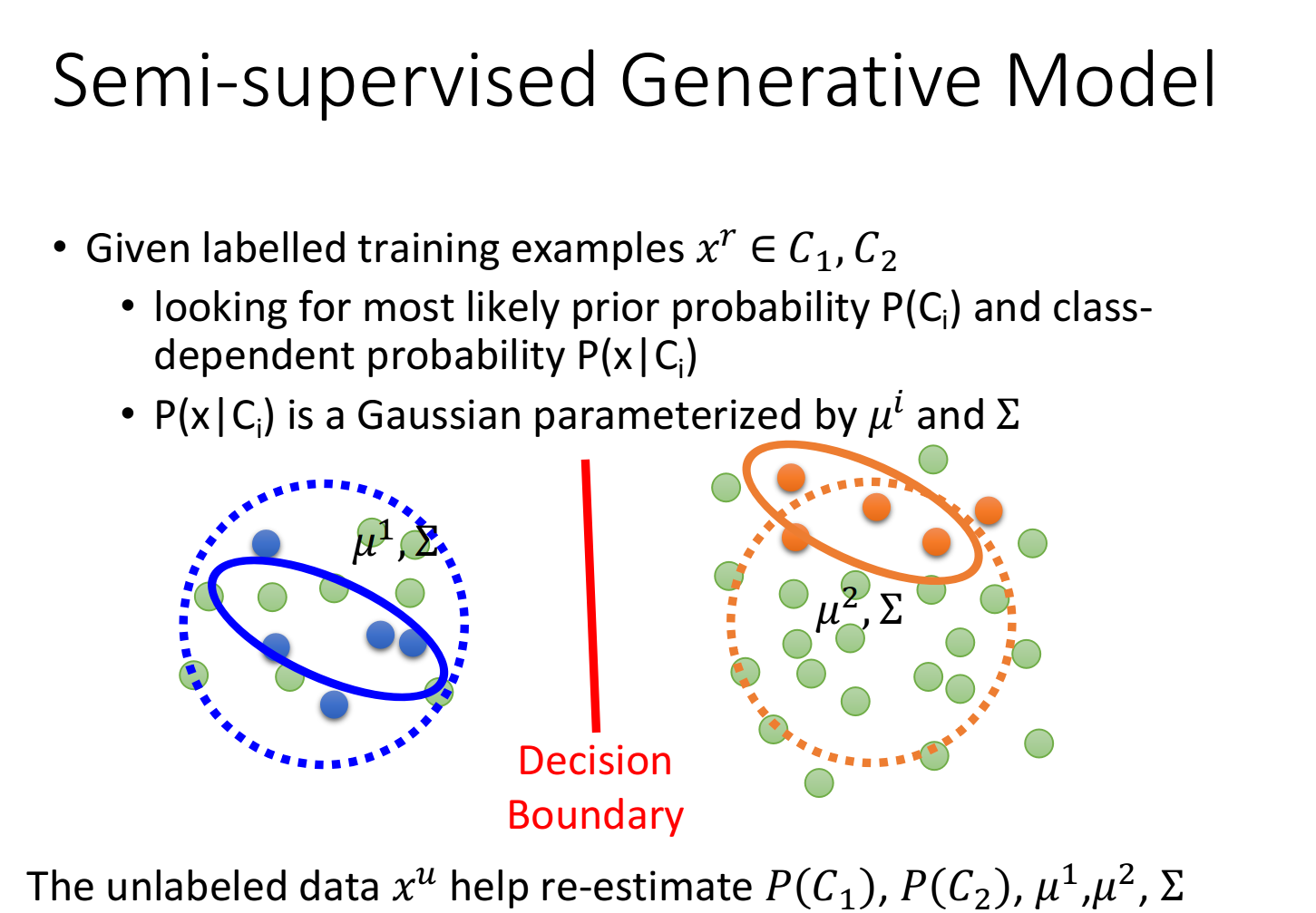• 先随机初始化一组参数： θ = P ( C 1 ) , P ( C 2 ) , u 1 , u 2 \theta={P(C_1),P(C_2),u^1,u^2}
• step1：利用初始化的model计算每一笔unlabeled data属于class1的概率，即 P θ ( C 1 ∣ x u ) P_{\theta}(C_1|x^u)
• step2：update model
考虑unlabeled data时，先验概率为
P ( C 1 ) = N 1 + ∑ x u P ( C 1 ∣ x u ) N P(C_1)=\frac{N_1+\sum_{x^u}P(C_1|x^u)}{N}
同理，对于均值为
u 1 = 1 N 1 ∑ x r ∈ C 1 x r + 1 ∑ x u P ( C 1 ∣ x u ) ∑ x u P ( C 1 ∣ x u ) x u u_1=\frac{1}{N_1}\sum\limits_{x^r \in {C_1}}x^r+\frac{1}{\sum_{x^u}P(C_1|x^u)}\sum\limits_{x^u}P(C_1|x^u)x^u
于是就有了一组新的参数 θ ′ \theta'
• 循环step1—step2
• 上述的step1就是EM algorithm里的E，step2则是M

P θ ( x u ) = P θ ( x u ∣ C 1 ) P ( C 1 ) + P θ ( x u ∣ C 2 ) P ( C 2 ) P_{\theta}(x^u)=P_{\theta}(x^u|C_1)P(C_1)+P_{\theta}(x^u|C_2)P(C_2)

l o g L ( θ ) = ∑ x r l o g P θ ( x r ) + ∑ x u l o g P θ ( x u ) logL(\theta)=\sum\limits_{x^r}logP_{\theta}(x^r)+\sum\limits_{x^u}logP_{\theta}(x^u)

### Low-density Separation Assumption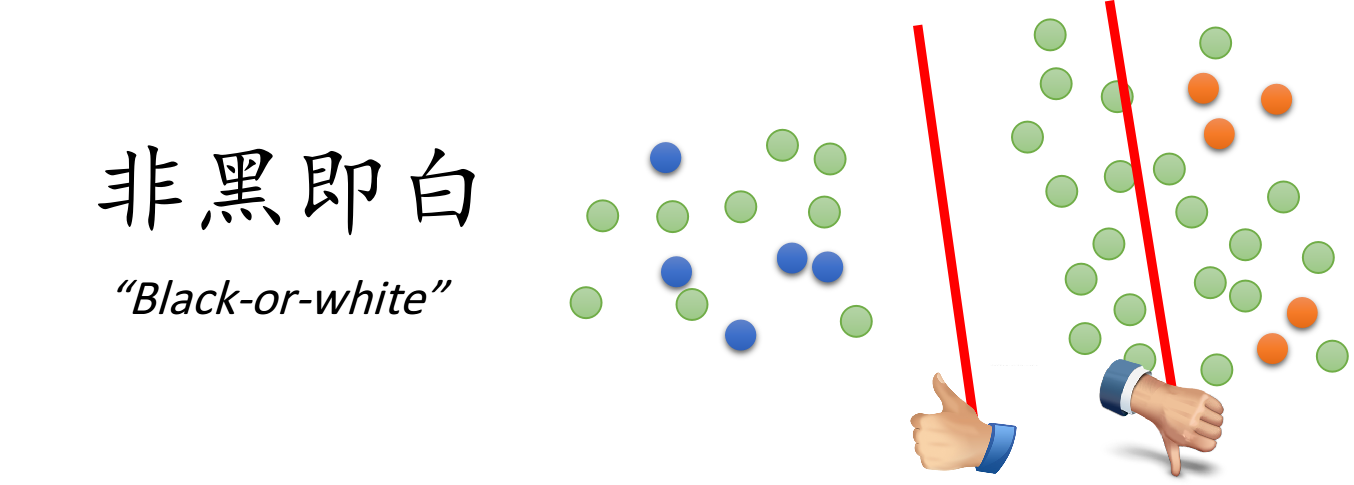#### Self Training

low-density separation最具代表性也最简单的方法是self training

• 先从labeled data训练一个model f ∗ f^*
• f ∗ f^* 对unlabeled data进行标记，被标记的data称为pseudo label（伪标签）， y u = f ∗ ( x u ) y^u=f^*(x^u)
• 从unlabeled data中拿一些data加到labeled data中
• 再训练model f ∗ f^* ，循环往复

• Self Training使用的是hard label：假设一笔data强制属于某个class
• Generative Model使用的是soft label：假设一笔data可以按照概率划分，不同部分属于不同class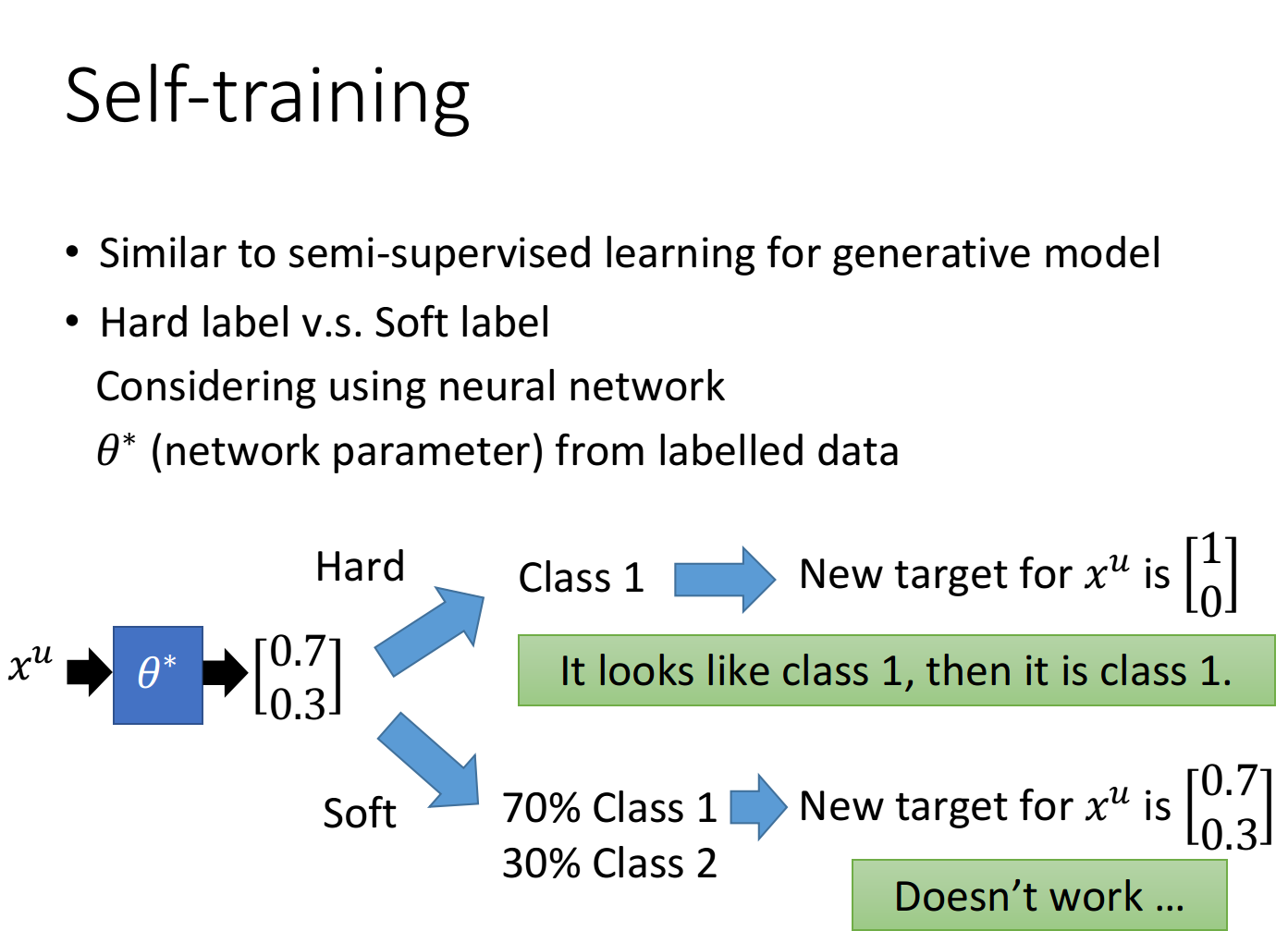#### Entropy-based Regularization

unlabeled data 的label到底是什么，但如果通过entropy-based regularization得到的分布集中在某个class上的话，那这个model就是好的，而如果分布是比较分散的，那这个model就是不好的，如下图所示：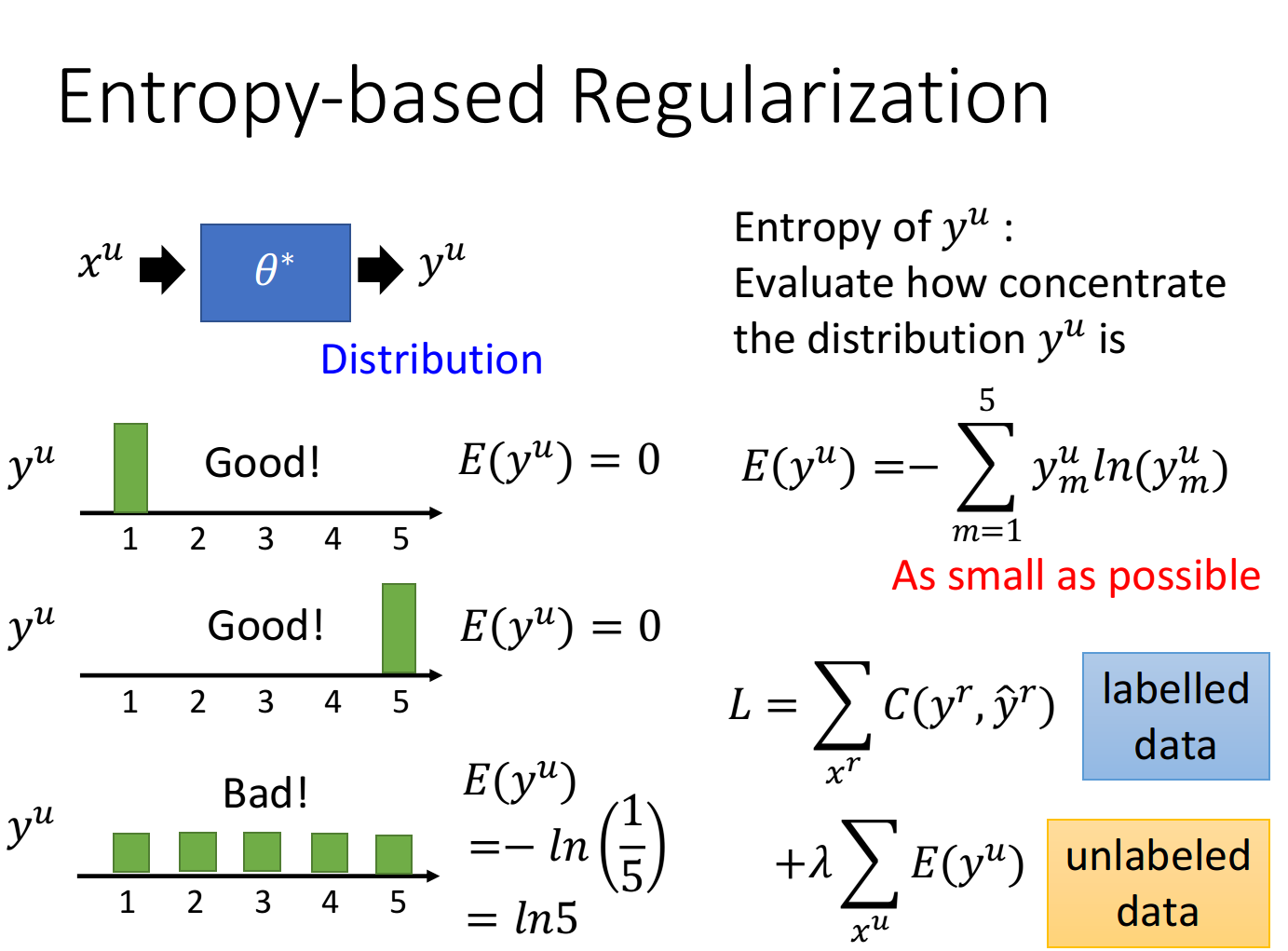E ( y u ) = − ∑ m = 1 5 y m u l n ( y m u ) E(y^u)=-\sum\limits_{m=1}^5y_m^uln(y^u_m)

entropy越大，distribution就越分散，entropy越小，distribution就越集中

• 对labeled data来说，它的output要跟正确的label越接近越好，用cross entropy表示如下：
L = ∑ x r C ( y r , y r ^ ) L=\sum\limits_{x^r}C(y^r,\hat{y^r})
• 对unlabeled data来说，要使得该distribution(也就是output)的entropy越小越好：
L = ∑ x r E ( y u ) L=\sum\limits_{x^r}E(y^u)
• 两项综合起来，可以用weight来加权，以决定哪个部分更为重要一些

#### Semi-supervised SVM

• 要有最大的margin，让这两个class分的越开越好
• 要分类错误越少越好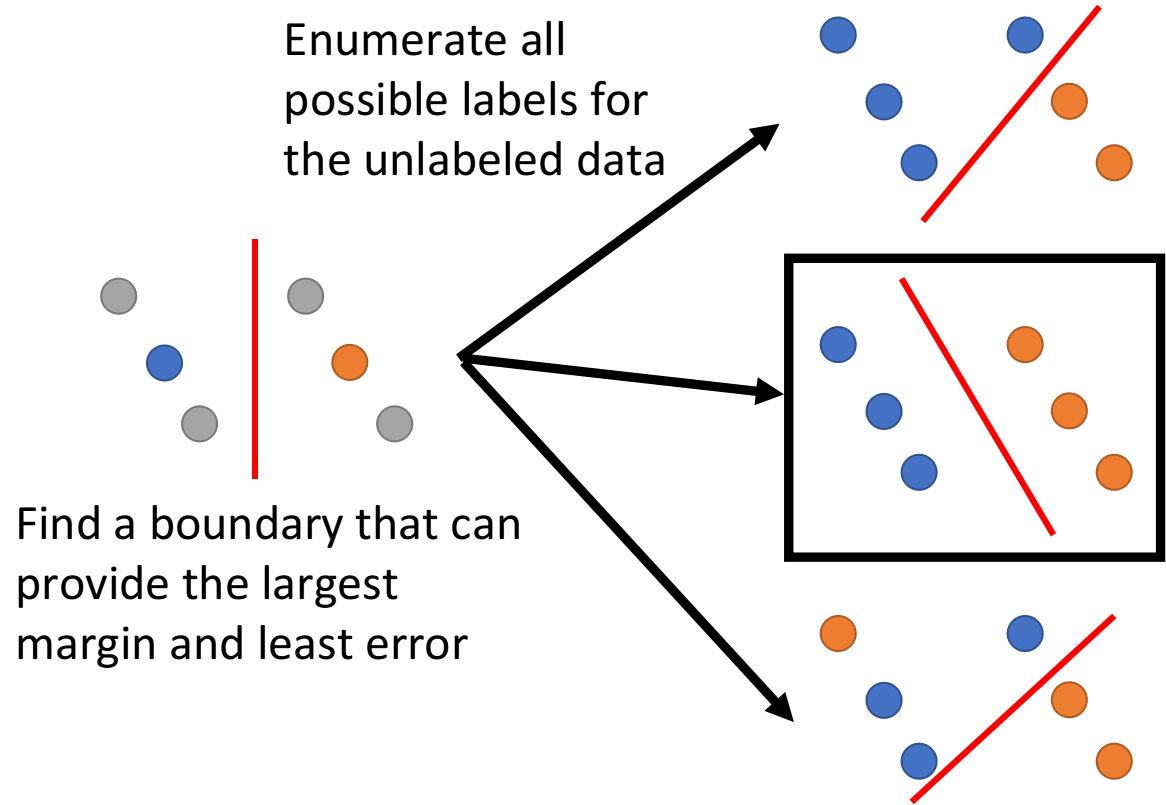### Smoothness Assumption

#### concepts

smoothness assumption的基本精神是：近朱者赤，近墨者黑

• x的分布是不平均的
• 如果 x 1 x^1 x 2 x^2 在一个high density region上很接近的话，那么 y 1 ^ \hat{y^1} y 2 ^ \hat{y^2} 是相同的
也就是这两个点可以在样本点高密度集中分布的区域块中有一条可连接的路径，即 connected by a high density path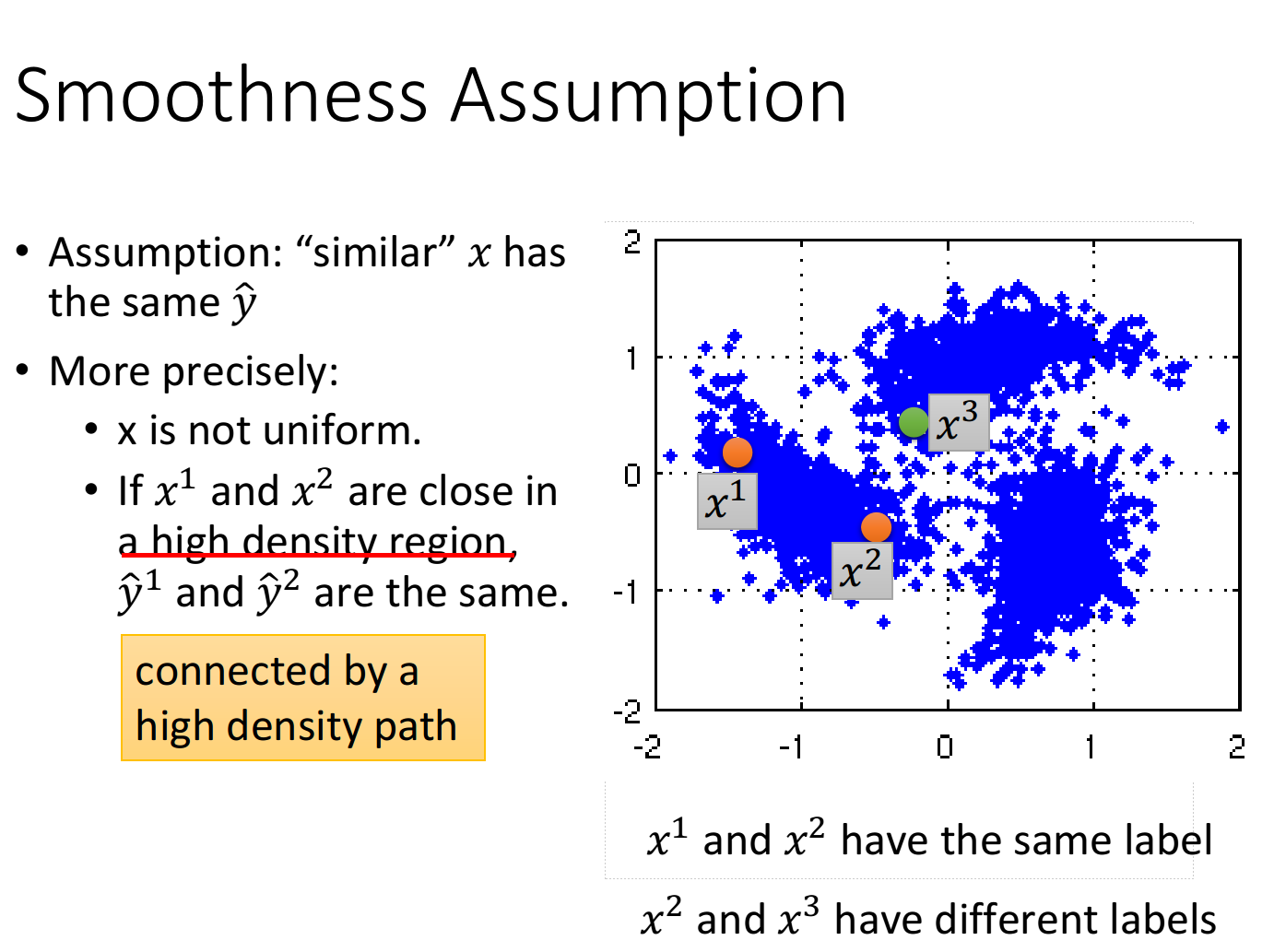#### digits detection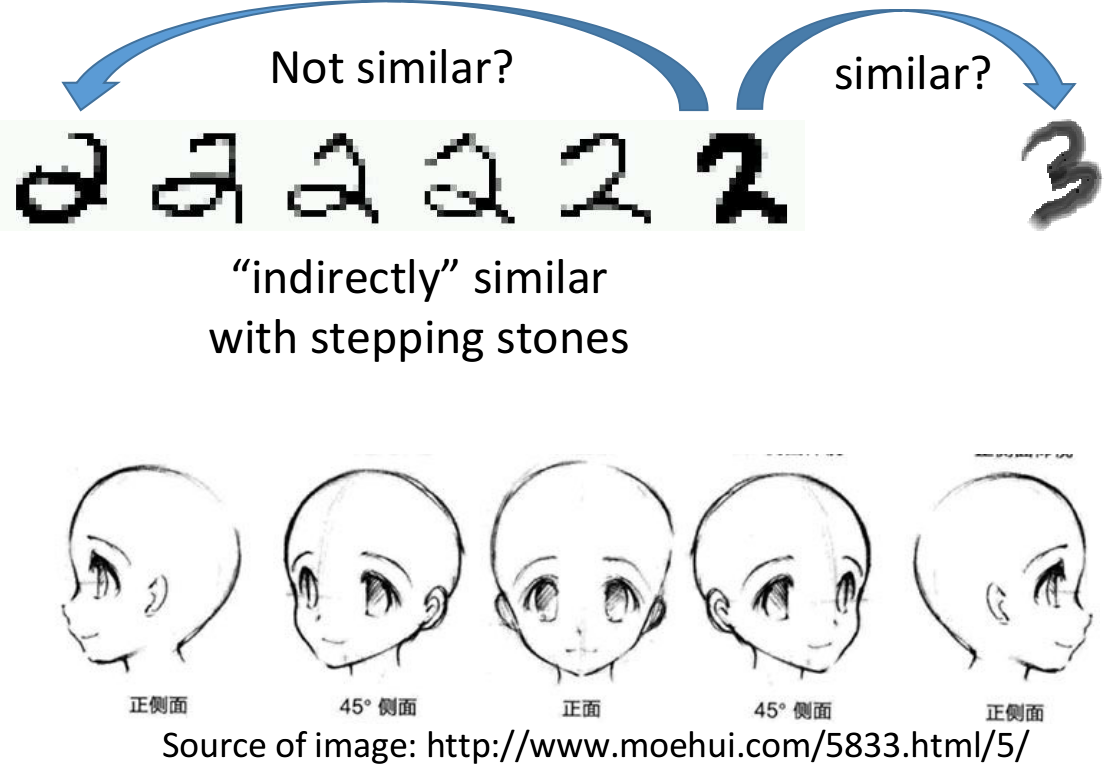#### file classification

Smoothness Assumption在文件分类上是非常有用的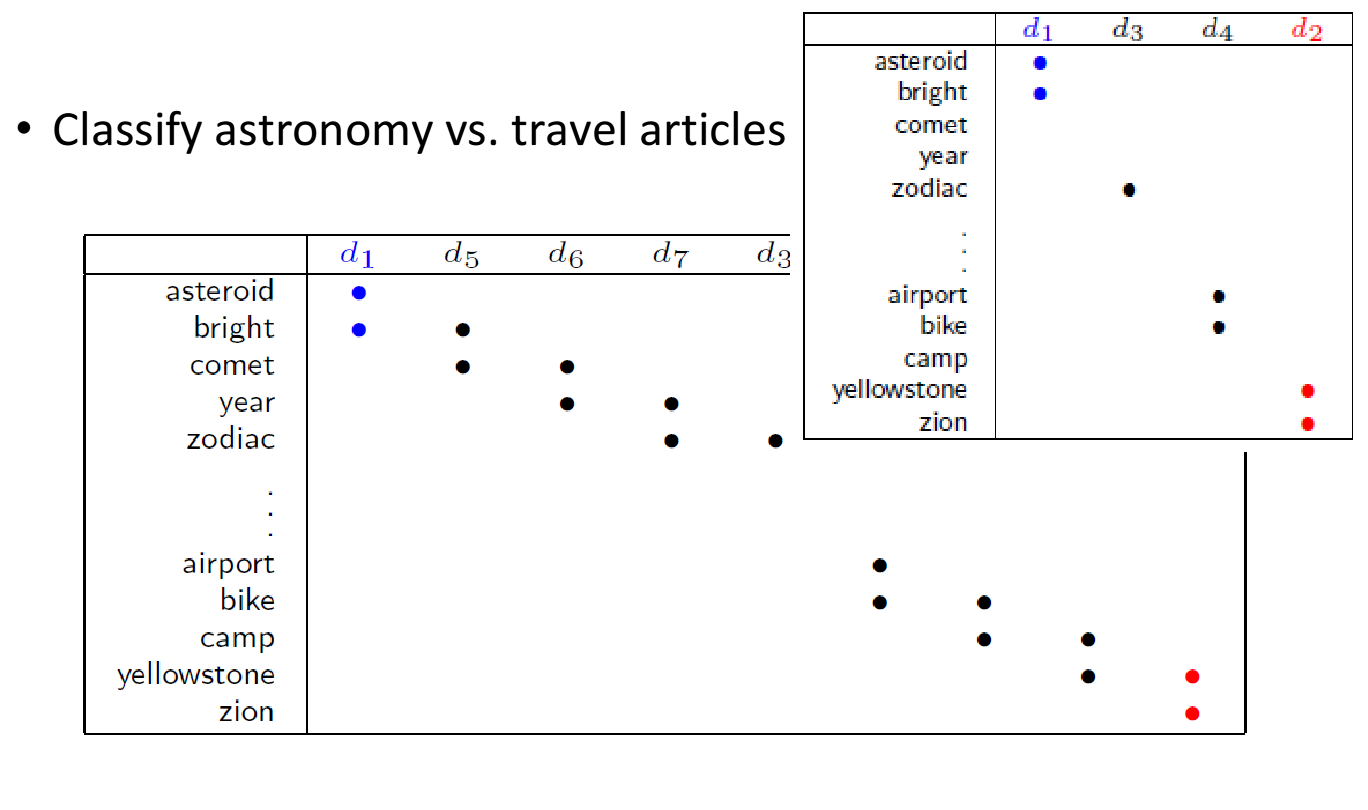#### cluster and then label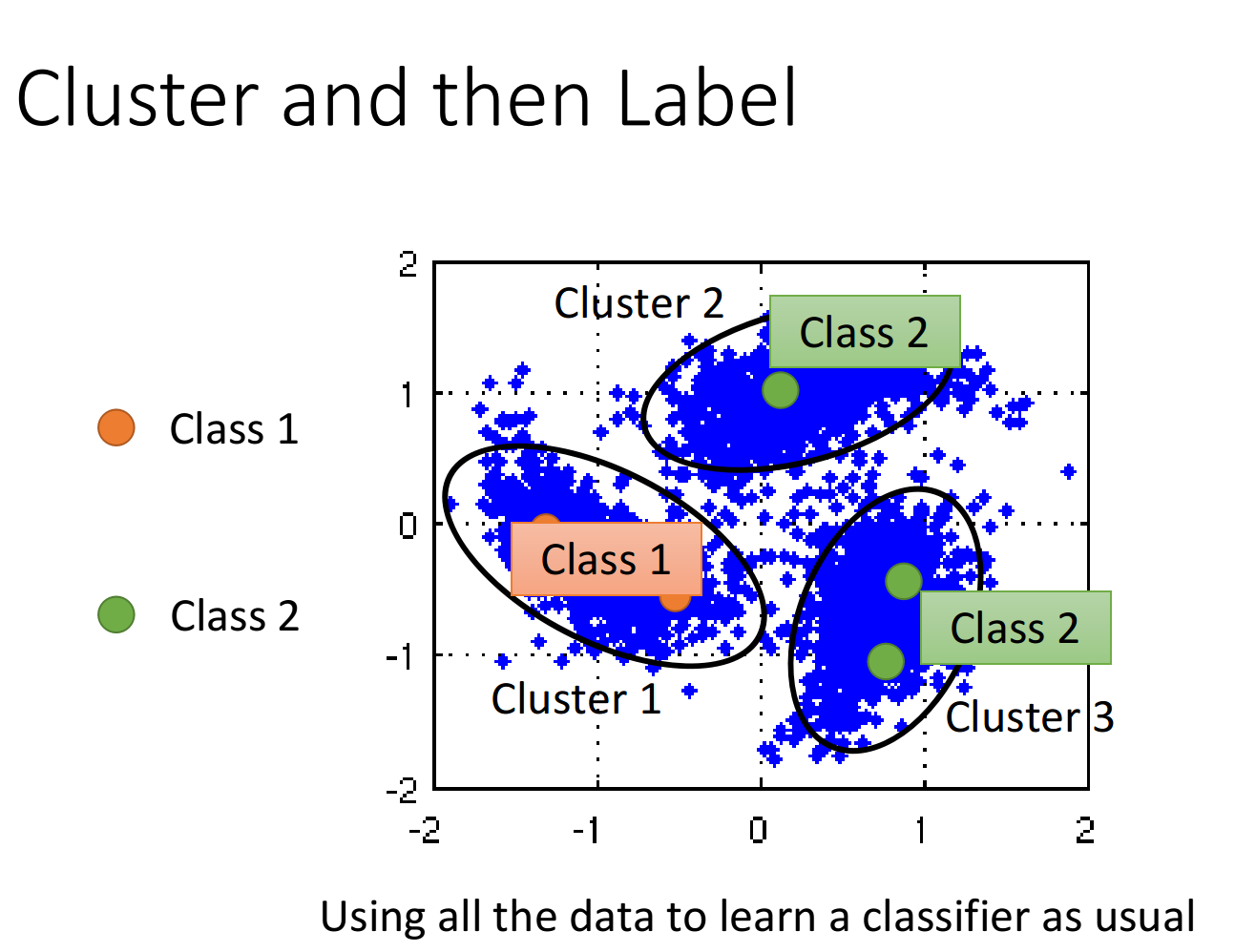#### Graph-based Approach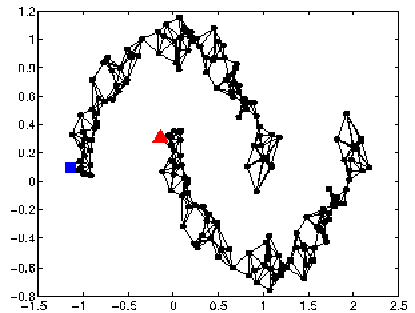graph的好坏，对结果起着至关重要的影响，而如何build graph却是一件heuristic的事情，需要凭着经验和直觉来做

• 首先定义两个object x i , x j x^i,x^j 之间的相似度 s ( x i , x j ) s(x^i,x^j)
如果是基于pixel的相似度，performance可能会不太好；建议使用autoencoder提取出来的feature来计算相似度，得到的performance会好一些
• 算完相似度后，就可以建graph了，方式有很多种：
k nearest neighbor：假设k=3，则每个point与相似度最接近的3个点相连
e-neighborhood：每个point与相似度超过某个特定threshold e的点相连
• 还可以给Edge特定的weight，让它与相似度 s ( x i , x j ) s(x^i,x^j) 成正比
1 建议用RBM function来确定相似度： s ( x i , x y ) = e ζ ∣ ∣ x i , x j ∣ ∣ 2 s(x^i,x^y)=e^{\zeta||x^i,x^j||^2}
计算两个vector的Euclidean Distance(欧几里得距离)，加上参数后再去exponential
2 至于加exponential，经验上来说通常是可以帮助提升performance的，在这里只有当 x i , x j x^i,x^j 非常接近的时候，singularity才会大；只要距离稍微远一点，singularity就会下降得很快，变得很小
3 使用exponential的RBM function可以做到只有非常近的两个点才能相连，稍微远一点就无法相连的效果，避免了下图中跨区域相连的情况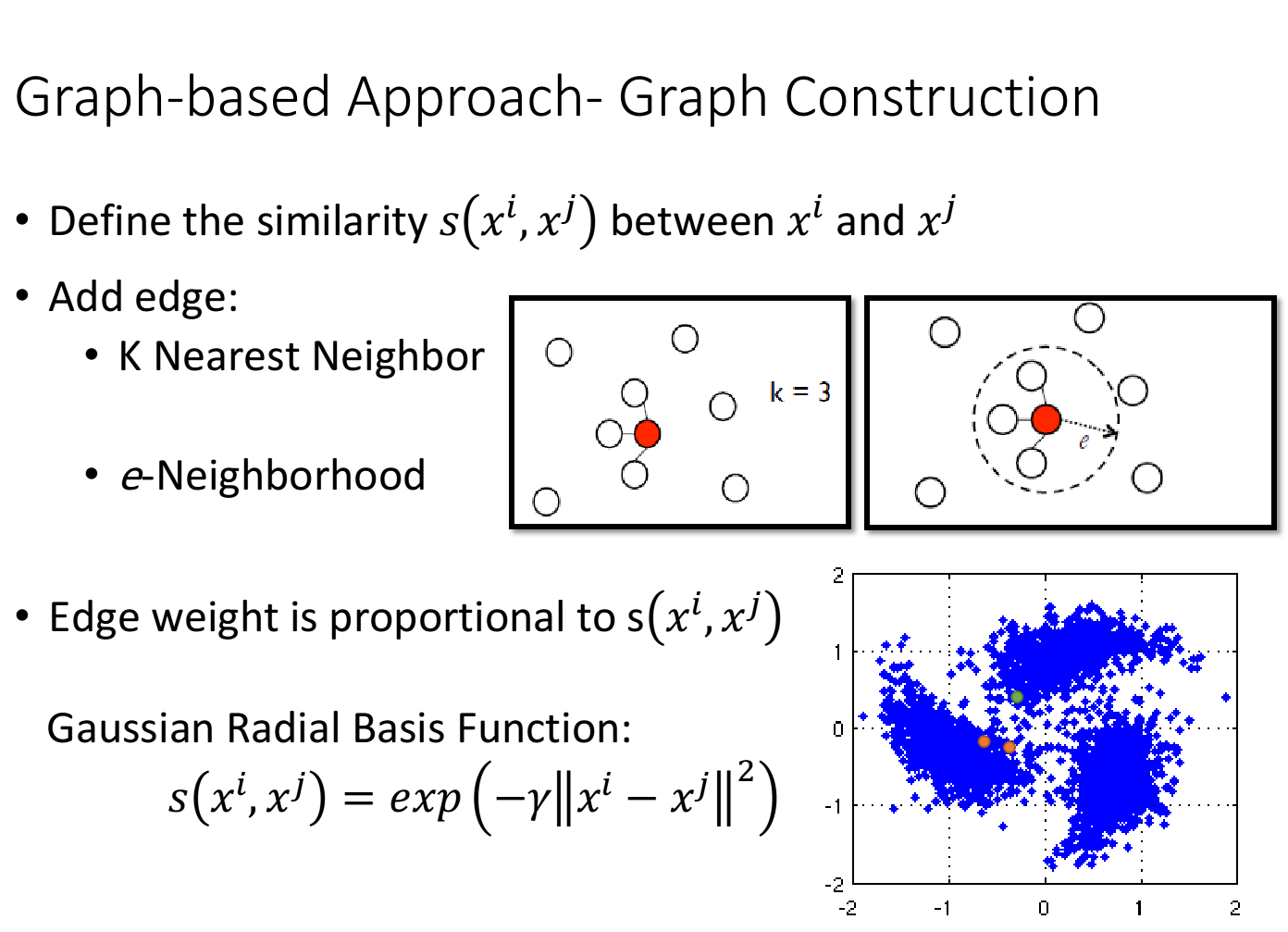graph-based approach的基本精神是，在graph上已经有一些labeled data，那么跟它们相连的point，属于同一类的概率就会上升，每一笔data都会去影响它的邻居，而graph带来的最重要的好处是，这个影响是会随着edges传递出去的，即使有些点并没有真的跟labeled data相连，也可以被传递到相应的属性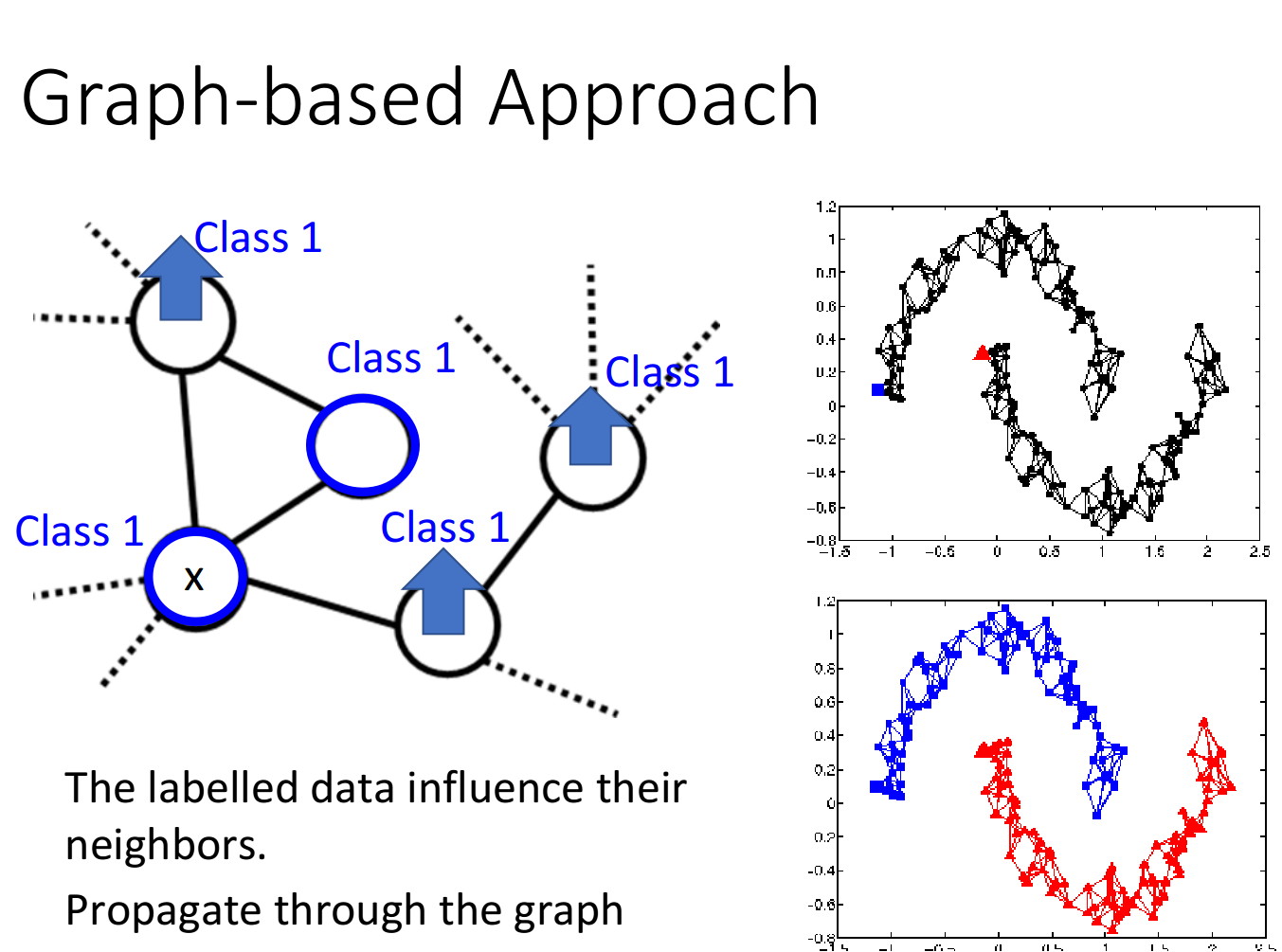S = 1 2 ∑ i , j w i , j ( y i − y j ) 2 S=\frac{1}{2}\sum\limits_{i,j}w_{i,j}(y^i-y^j)^2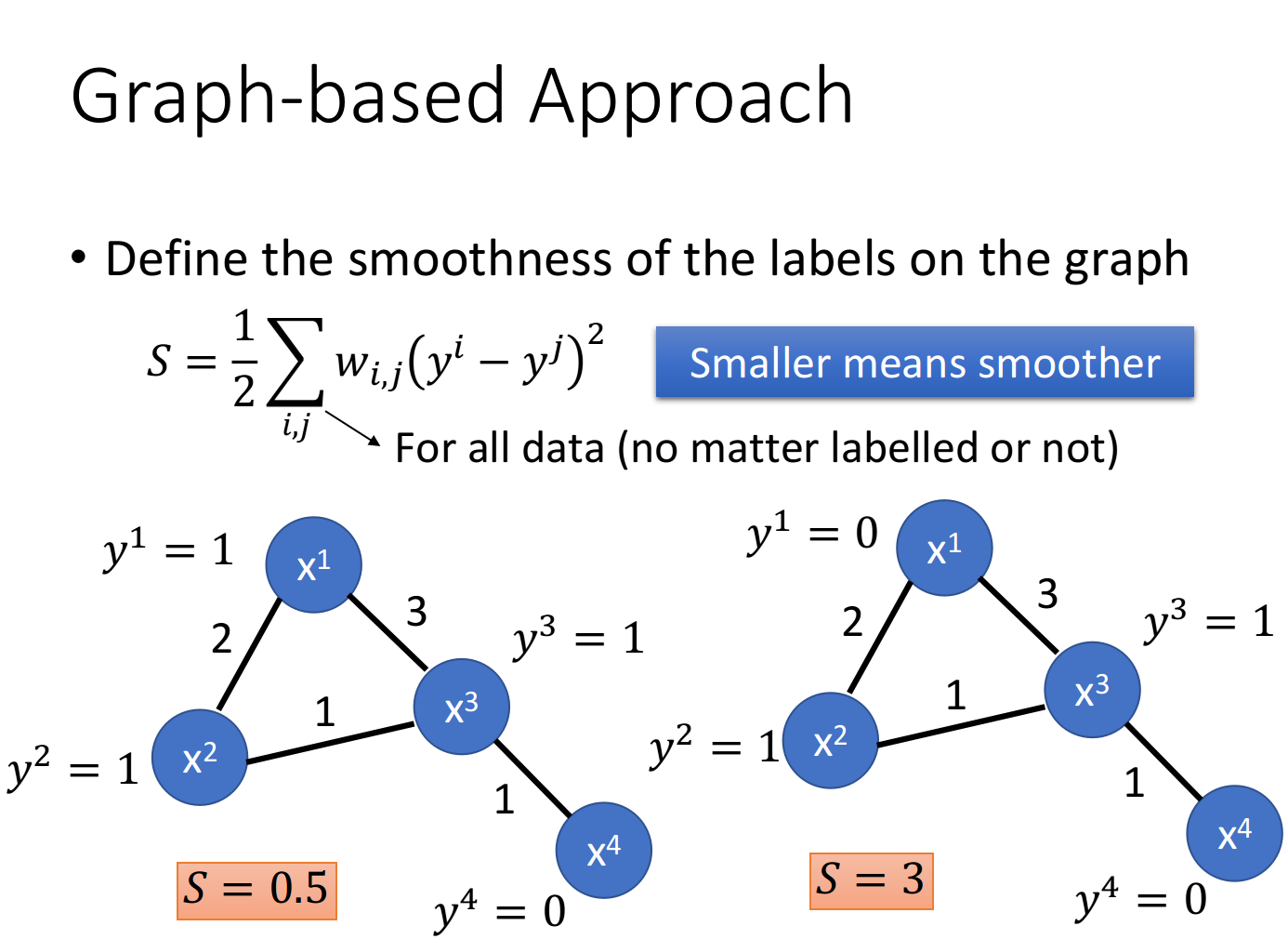y = [ . . . y i . . . y j ] T y=[...y^i...y^j]^T

S = 1 2 ∑ i , j w i , j ( y i − y j ) 2 = y T L y S=\frac{1}{2}\sum\limits_{i,j}w_{i,j}(y^i-y^j)^2=y^TLy

• W：把data point两两之间weight的关系建成matrix，代表了 x i x^i x j x^j 之间的weight值
• D：把W的每一个row上的值加起来放在该行对应的diagonal上即可，比如5=2+3,3=2+1,…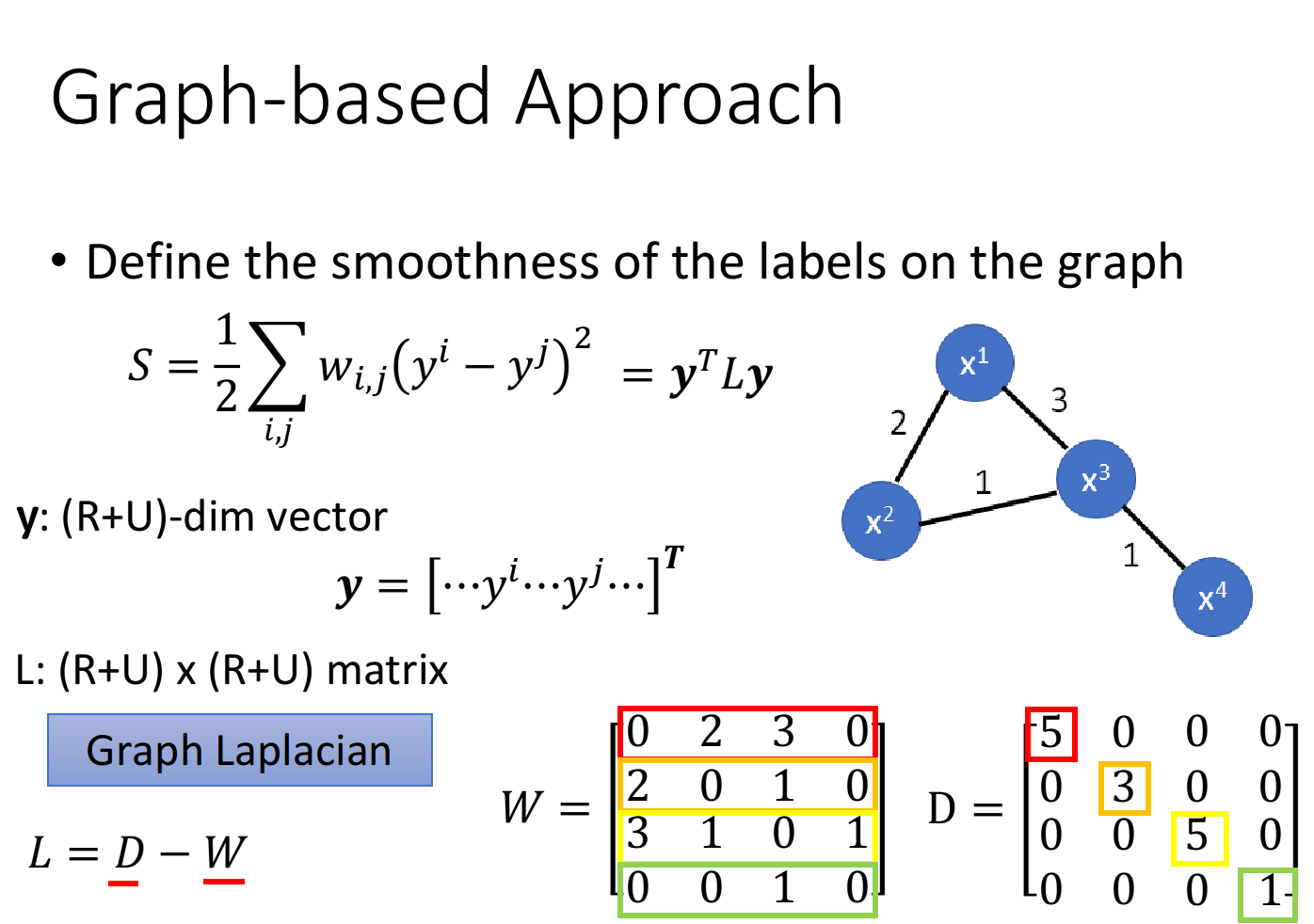S = y T L y S=y^TLy 来说，y是label，是neural network的output，取决于neural network的parameters，因此要在原来仅针对labeled data的loss function中加上这一项，得到：

L = ∑ x r C ( y r , y ^ r ) + λ S L=\sum\limits_{x^r}C(y^r,\hat{y}^r)+\lambda S

λ S \lambda S 实际上也是一个regularization term

• labeled data的cross entropy越小越好(neural network的output跟真正的label越接近越好)
• smooth S越小越好(neural network的output，不管是labeled还是unlabeled，都要符合Smoothness Assumption的假设)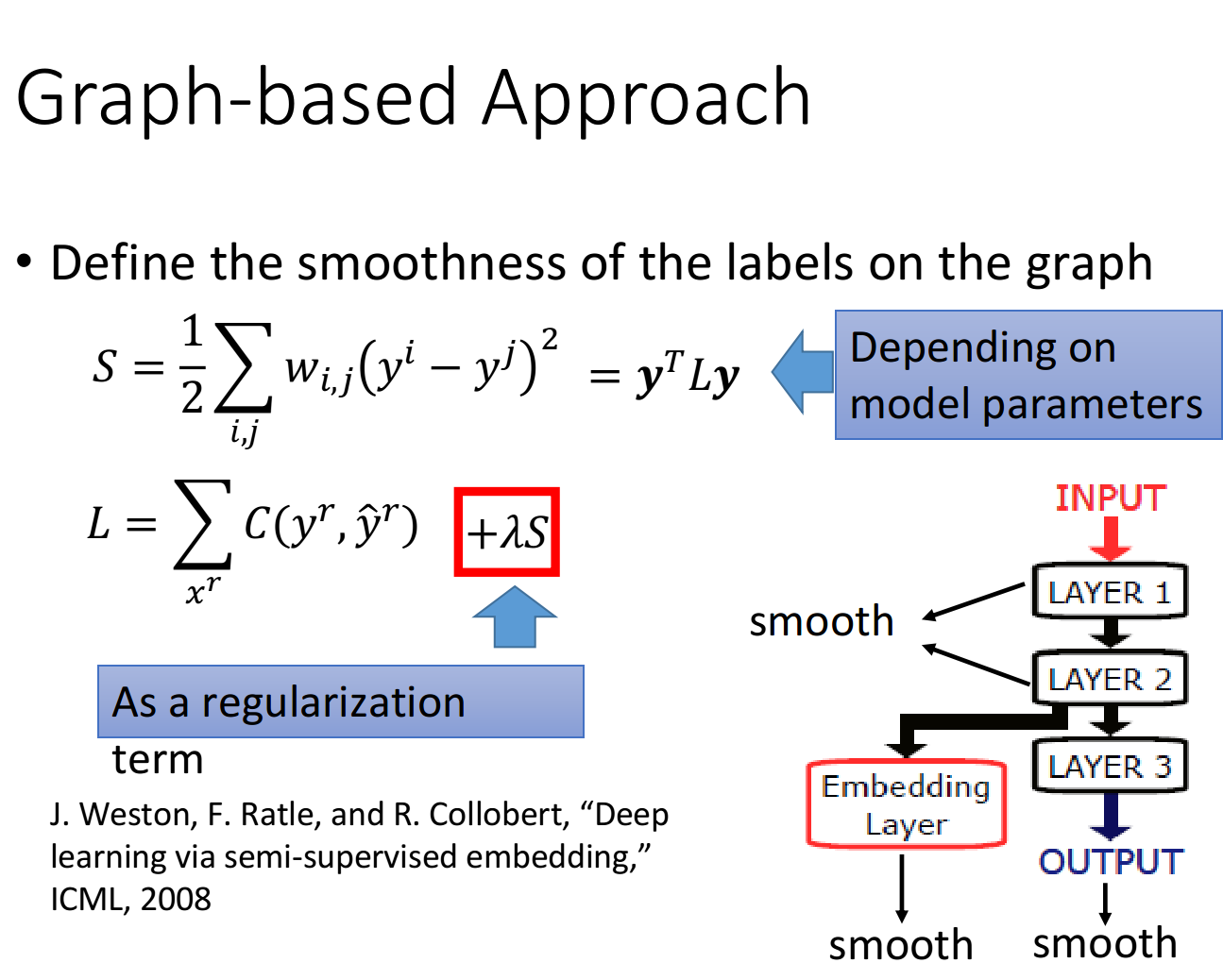### Better Representation

Better Representation的精神是，去芜存菁，化繁为简

### Reference

https://sakura-gh.github.io/ML-notes/ML-notes-html/15_Semi-supervised-Learning.html### 低价透明### 金牌服务### 信息保密